Author: Oscar Cronquist Article last updated on March 19, 2021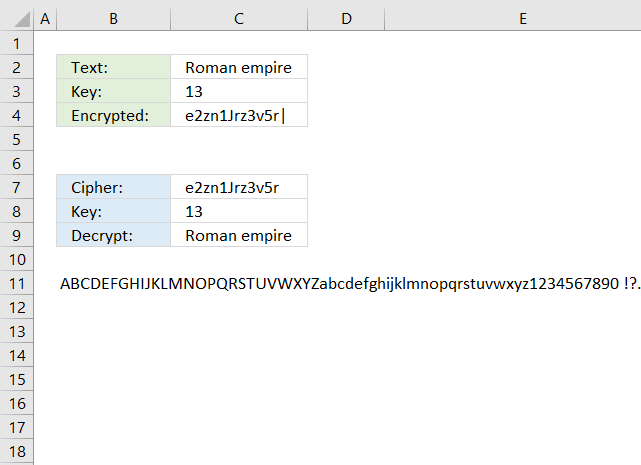Most if not all formulas presented here use functions that are only available in Excel 365. Some but perhaps not all formulas can be converted so they can be used in older Excel versions. The TEXTJOIN function requires a VBA user defined function which you can find here: How to use the TEXTJOIN function

Each section contains a formula that encrypts plain text messages and a formula that decrypts a cipher. Often the encrypted text is used to decrypt it back to plain text to make sure that the decryption formula works fine.

## 1. Reverse text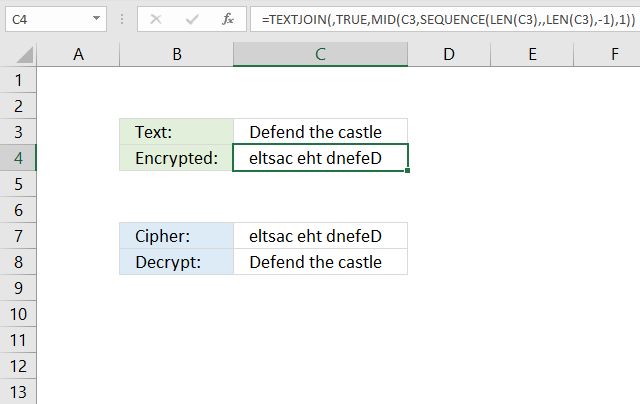The formula in cell C4 changes the text entered in cell C3, the last character is first and the first character is last, and so on. This simple step can make it harder for a dictionary attack to recognize words.

Formula in cell C4:

=TEXTJOIN(, TRUE, MID(C3, SEQUENCE(LEN(C3), , LEN(C3), -1), 1))

Formula in cell C8:

=TEXTJOIN(, TRUE, MID(C7, SEQUENCE(LEN(C7), , LEN(C7), -1), 1))

## 2. Insert random characters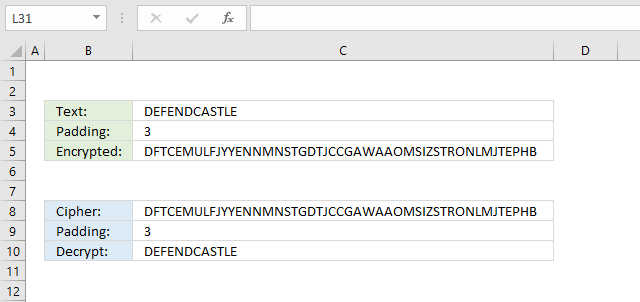The formula in cell C4 inserts random characters between each plaintext character based on the number in cell C4.

Formula in cell C4:

=UPPER(TEXTJOIN(, FALSE, MID(C3, SEQUENCE(LEN(C3)), 1)&MID(TEXTJOIN(, TRUE, CHAR(RANDARRAY(LEN(C3)*C4, , 65, 90, TRUE))), SEQUENCE(LEN(C3), , 1, C4), C4)))

Formula in cell C8:

=TEXTJOIN(, FALSE, MID(C8, SEQUENCE(LEN(C8)/2, , , C9+1), 1))

## 3. Convert letters to numbers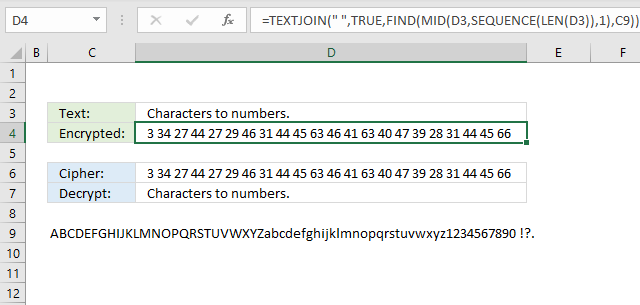The formula in cell D4 converts each letter in cell D3 to a number representing the relative position in the alphabet displayed in cell C9. You can easily randomize the alphabet to make the encryption even harder.

There is a formula in the next section that demonstrates how to pseudo-randomize characters in a given text string.

This is a simple substitution cipher and can easily be decrypted using frequency analysis.

Formula in cell C4:

=TEXTJOIN(" ", TRUE, FIND(MID(D3, SEQUENCE(LEN(D3)), 1), C9))

Formula in cell C8:

=TEXTJOIN(, TRUE, MID(C9, TRIM(MID(SUBSTITUTE(\$D6, " ", REPT(" ", 99)), SEQUENCE(LEN(D6)-LEN(SUBSTITUTE(D6, " ", ""))+1)*99-98, 99)), 1))

## 4. How to shuffle characters in the alphabet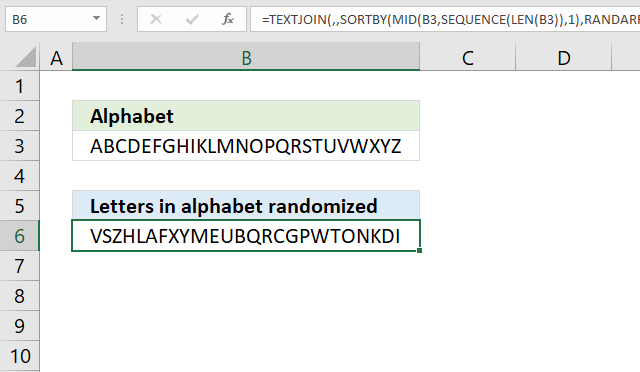The formula in cell B6 changes the order of each character in cell B3. Press F9 to recalculate.

Formula in cell B6:

=TEXTJOIN(, , SORTBY(MID(B3, SEQUENCE(LEN(B3)), 1), RANDARRAY(LEN(B3))))

## 5. Convert string to HEX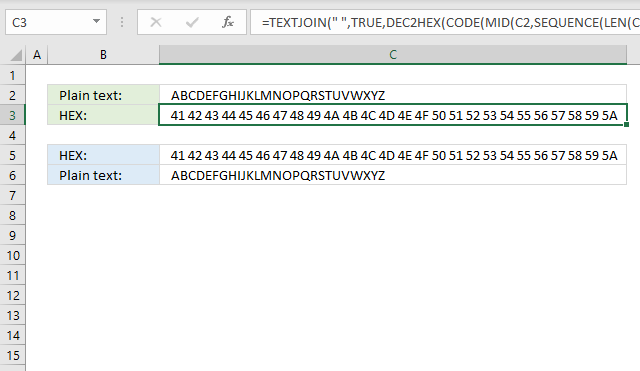The formula in cell C3 extracts each character, converts them to their ASCII code, and then calculates the corresponding hexadecimal value.

Formula in cell C3:

=TEXTJOIN(, TRUE, DEC2HEX(CODE(MID(C2, SEQUENCE(LEN(C2)), 1))))

Formula in cell C6:

=TEXTJOIN(, TRUE, CHAR(HEX2DEC(MID(C4, SEQUENCE(LEN(C4)/2, , , 2), 2))))

## 6. Convert string to ASCIIThe image above demonstrates a formula that converst letters to their corresponding ASCII code number. This makes it a simple substitution cipher.

Formula in cell C3:

=TEXTJOIN(, TRUE, CODE(MID(C2, SEQUENCE(LEN(C2)), 1)))

Formula in cell C6:

=TEXTJOIN(, TRUE, CHAR(MID(C4, SEQUENCE(LEN(C4)/2, , , 2), 2)))

## 7. Convert string to Base64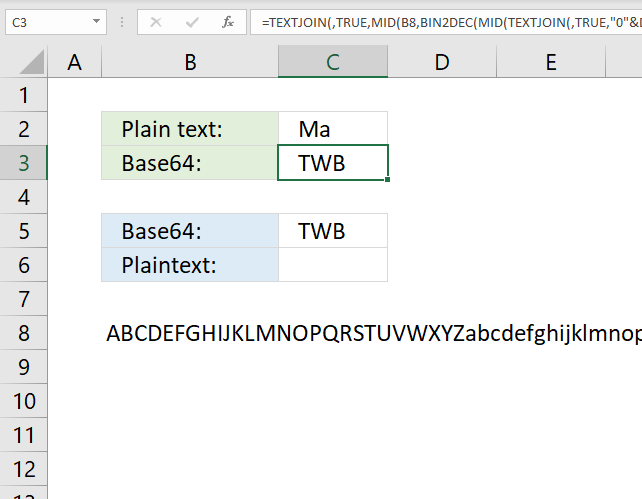The formula in cell C3 converts a string entered in cell C2 and returns base64, it works for strings longer than or equal to 2 characters. Note, Base64 is not a cipher.

Formula in cell C3:

=TEXTJOIN(, TRUE, MID(B8, BIN2DEC(MID(TEXTJOIN(, TRUE, "0"&DEC2BIN(CODE(MID(C2, SEQUENCE(LEN(C2)), 1)))), SEQUENCE(ROUNDUP(SUM(LEN("0"&DEC2BIN(CODE(MID(C2, SEQUENCE(LEN(C2)), 1)))))/6, 0), , , 6), 6))+1, 1))

The equal sign is sometimes used as padding, however, the formula above does not append the character automatically.

## 8. Ceasar cipherThe Caesar cipher is a simple substitution cipher that rotates the alphabet based on the number in cell C3. A becomes n and so on. Read more: Caeser cipher

The English alphabet has 26 letters, if you use 13 as a key you can use the same calculation to both encrypt and decrypt. ROT13 is a Caesar cipher with 13 as a key. The formulas shown here works for all key numbers.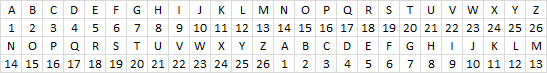You can change the alphabet used by editing the string in cell B11. For example, use only uppercase letters and the alphabet becomes ABCDEFGHIJKLMNOPQRSTUVWXYZ.

Formula in cell C4:

=TEXTJOIN(, TRUE, MID(B11, MOD(FIND(MID(C2, SEQUENCE(LEN(C2)), 1), B11)-1+C3, LEN(B11))+1, 1))&"|"

Formula in cell C9:

=TEXTJOIN(, TRUE, MID(B11, MOD(FIND(MID(C7, SEQUENCE(LEN(C7)), 1), B11)-1-C8, LEN(B11))+1, 1))

## 9. Atbash cipher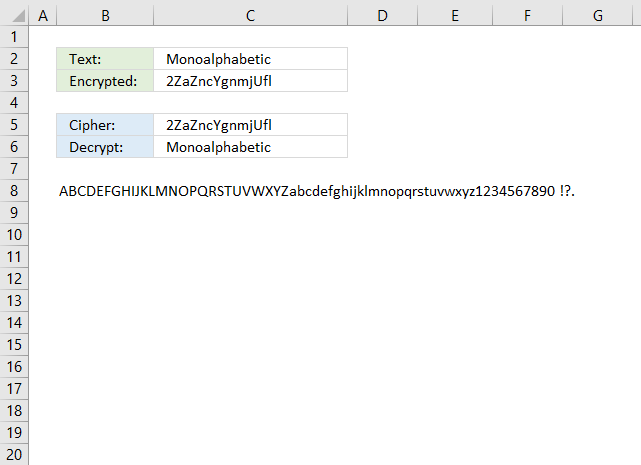The Atbash cipher is a simple substitution cipher that maps each character to its reverse. The first letter becomes the last and the second letter becomes the second last letter and so on.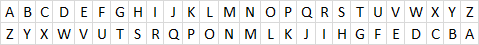The example shown in the image above uses both upper and lower letters including numbers and some other characters. Change the value in cell B8 to ABCDEFGIJKLMNOPQRSTUVWXYZ if you want an easier alphabet.

Formula in cell C3:

=TEXTJOIN(, FALSE, MID(B8, LEN(B8)-FIND(MID(C2, SEQUENCE(LEN(C2)), 1), B8)+1, 1))

Formula in cell C6:

=TEXTJOIN(, FALSE, MID(B8, LEN(B8)-FIND(MID(C5, SEQUENCE(LEN(C5)), 1), B8)+1, 1))

## 10. Trithemius cipher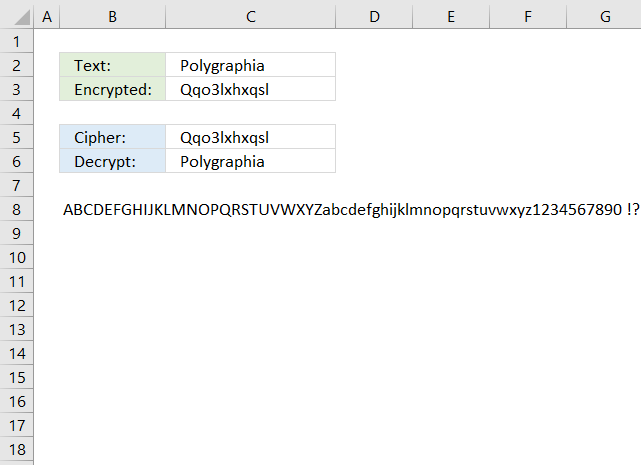Trithemius cipher moves one character by each step. This makes it much harder to crack using frequency analysis.

For example, ABC becomes BDF. A tabula recta shown below makes it easier to encrypt and decrypt strings manually using the Trithemius cipher, however, the formulas below makes it even easier. Simply type the plain text message in cell C2 and the formula in cell C3 encrypts it for you based on the alphabet provided in cell B8.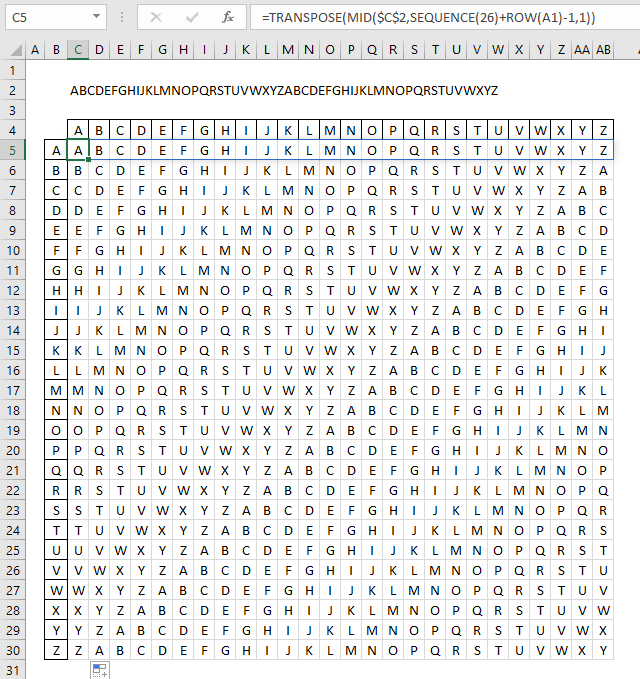Formula in cell C3:

=TEXTJOIN(, TRUE, MID(B8, MOD(FIND(MID(C2, SEQUENCE(LEN(C2)), 1), B8)+SEQUENCE(LEN(C2))-1, LEN(B8))+1, 1))

Formula in cell C6:

=TEXTJOIN(, TRUE, MID(B8, MOD(FIND(MID(C5, SEQUENCE(LEN(C5)), 1), B8)-SEQUENCE(LEN(C5))-1, LEN(B8))+1, 1))

## 11. Substitution cipher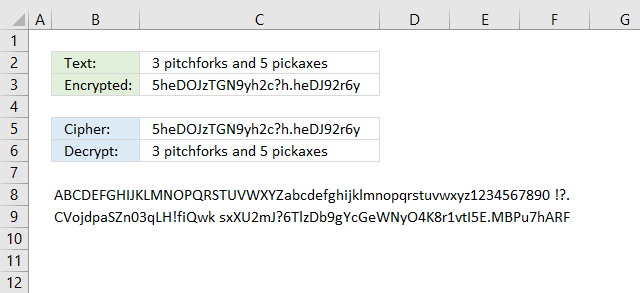The substitution cipher maps each character to a different character. Use frequency analysis to break the substitution cipher here is a frequency analysis formula.

The example above allows you to use whatever alphabet you want and map it to whatever character you want. I am using both upper and lower letters, as well as, numbers and some other often used characters.

Formula in cell C3:

=TEXTJOIN(, FALSE, MID(B9, FIND(MID(C2, SEQUENCE(LEN(C2)), 1), B8), 1))

Formula in cell C6:

=TEXTJOIN(, FALSE, MID(B8, FIND(MID(C5, SEQUENCE(LEN(C5)), 1), B9), 1))

A twitter account belonging to NSA posted this weird message one morning in the beginning of May.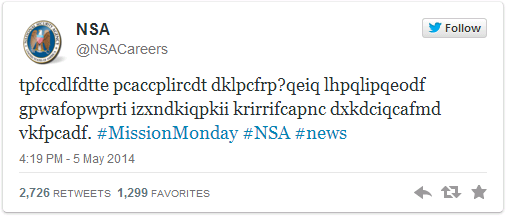It is a basic substitution cipher meaning t=w, p=a and so on. The message is "Want to know what it takes to work at NSA? Check back each Monday in May as we explore careers essential to protecting our nation"

A famous substitution cipher is the Caesar cipher, rotating each letter a number of places.According to wikipedia, the cipher was reasonably secure at the time because Caesar's enemies would have been illiterate. :-)

The following macro rotates each letter in cell B2 by a number found in cell B8.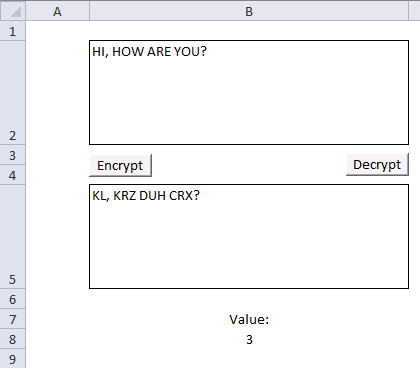VBA Code

```Sub Encrypt()
Dim enc As String
Dim res As String

enc = Range("B2")
For i = 1 To Len(enc)
If Asc(UCase(Mid(enc, i, 1))) &amp;amp;amp;amp;amp;amp;amp;amp;amp;amp;lt; 91 And Asc(UCase(Mid(enc, i, 1))) &amp;amp;amp;amp;amp;amp;amp;amp;amp;amp;gt; 64 Then
If (Asc(UCase(Mid(enc, i, 1))) + Range("B8")) &amp;amp;amp;amp;amp;amp;amp;amp;amp;amp;gt; 90 Then
res = res &amp;amp;amp;amp;amp;amp;amp;amp;amp;amp;amp; Chr(65 + (Asc(UCase(Mid(enc, i, 1))) + Range("B8") - 90))
ElseIf (Asc(UCase(Mid(enc, i, 1))) + Range("B8")) &amp;amp;amp;amp;amp;amp;amp;amp;amp;amp;lt; 65 Then
res = res &amp;amp;amp;amp;amp;amp;amp;amp;amp;amp;amp; Chr(90 - (64 - (Asc(UCase(Mid(enc, i, 1))) + Range("B8"))))
Else
res = res &amp;amp;amp;amp;amp;amp;amp;amp;amp;amp;amp; Chr(Asc(UCase(Mid(enc, i, 1))) + Range("B8"))
End If
Else
res = res &amp;amp;amp;amp;amp;amp;amp;amp;amp;amp;amp; Mid(enc, i, 1)
End If
Next i

Range("B5") = res

End Sub

Sub Decrypt()

Dim dec As String
Dim res As String
dec = Range("B5")
For i = 1 To Len(dec)
If Asc(UCase(Mid(dec, i, 1))) &amp;amp;amp;amp;amp;amp;amp;amp;amp;amp;lt; 91 And Asc(UCase(Mid(dec, i, 1))) &amp;amp;amp;amp;amp;amp;amp;amp;amp;amp;gt; 64 Then
If (Asc(UCase(Mid(dec, i, 1))) - Range("B8")) &amp;amp;amp;amp;amp;amp;amp;amp;amp;amp;gt; 90 Then
res = res &amp;amp;amp;amp;amp;amp;amp;amp;amp;amp;amp; Chr(65 + (Asc(UCase(Mid(dec, i, 1))) - Range("B8") - 91))
ElseIf (Asc(UCase(Mid(dec, i, 1))) - Range("B8")) &amp;amp;amp;amp;amp;amp;amp;amp;amp;amp;lt; 65 Then
res = res &amp;amp;amp;amp;amp;amp;amp;amp;amp;amp;amp; Chr(90 - (64 - (Asc(UCase(Mid(dec, i, 1))) - Range("B8"))))
Else
res = res &amp;amp;amp;amp;amp;amp;amp;amp;amp;amp;amp; Chr(Asc(UCase(Mid(dec, i, 1))) - Range("B8"))
End If
Else
res = res &amp;amp;amp;amp;amp;amp;amp;amp;amp;amp;amp; Mid(dec, i, 1)
End If
Next i

Range("B2") = res

End Sub
```

### Get excel *.xlsm file

Encrypt message.xlsm

## 12. Transposition cipher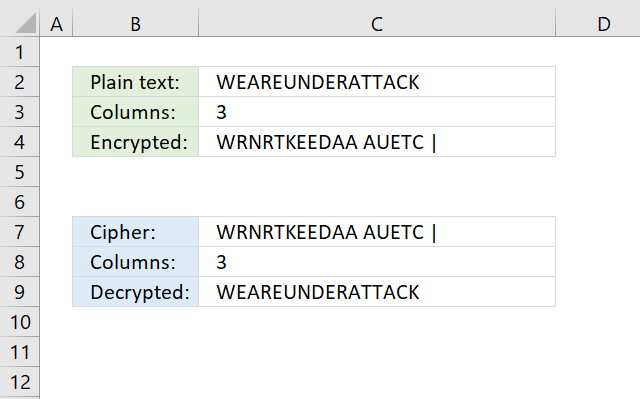The transposition cipher deploys characters in a grid based on the number of columns entered in cell C3.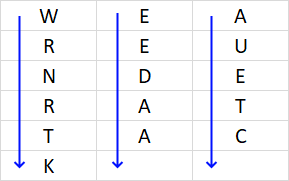Concatenate characters starting from the upper left corner moving down, then continue with the next column, and so on. The formula below adds a | character to show if the last character is the space character.

The transposition cipher can be broken using frequency analysis and anagramming.

Formula in cell C4:

=TEXTJOIN(, FALSE, TRANSPOSE(MID(C2&REPT(" ", C3-MOD(LEN(C2), C3)), SEQUENCE(ROUNDUP(LEN(C2)/C3,0), C3, 1), 1)))&"|"

The formula in cell C9 removes the last | character automatically. Change LEN(C7)-1 to Len(C7) to remove that functionality.

Formula in cell C9:

=TEXTJOIN(, FALSE, TRANSPOSE(MID(C7, SEQUENCE(C8, ROUNDUP((LEN(C7)-1)/C8, 0), 1), 1)))

## 13. Column transposition cipher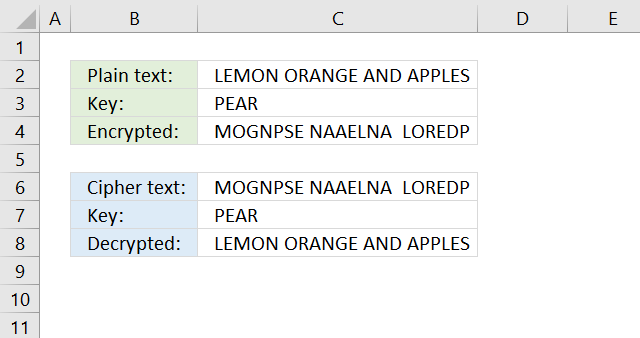The column transposition cipher uses a key to rearrange the columns in a given order based on the positions of the key characters in the alphabet.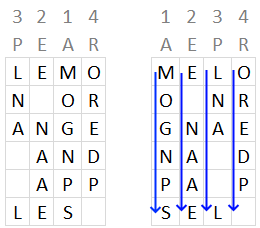Formula in cell C4:

=TEXTJOIN("", TRUE, SORTBY(TRANSPOSE(MID(C2, SEQUENCE(ROUNDUP(LEN(C2)/LEN(C3), 0), LEN(C3)), 1)), MID(C3, SEQUENCE(LEN(C3)), 1)))

Formula in cell C8:

=TEXTJOIN(, TRUE, TRANSPOSE(SORTBY(MID(C6, SEQUENCE(LEN(C7), ROUNDUP(LEN(C6)/LEN(C7), 0)), 1), MATCH(SORT(MID(C7, SEQUENCE(LEN(C7)), 1)), MID(C7, SEQUENCE(LEN(C7)), 1), 0))))

## 15. Vigenere cipher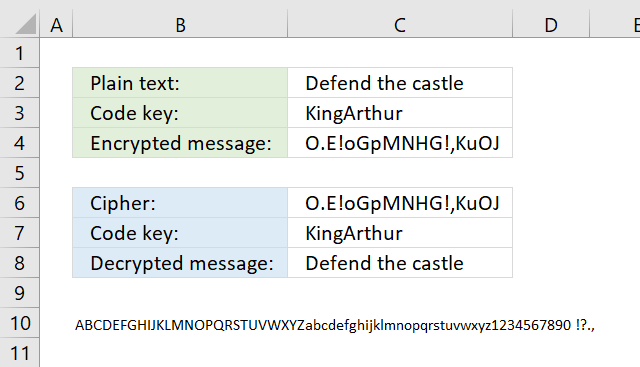The Vigenere cipher uses the same key repeatedly across the entire message, here is a detailed explanation: Vigenere cipher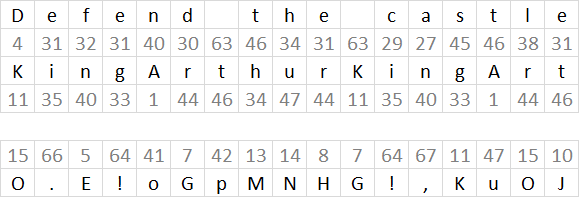The formulas finds the relative position of each character of both plain text message and the code key in the alphabet, provided in cell B10.

They then add the numbers, see image above. The sum is then the position of a different character in the alphabet.

Sometimes the sum is larger than the number of characters in the provided alphabet. The formulas calculate the remainder and use that number to extract a character.

You can change the alphabet in cell B10 to only upper letters if you want a more read-friendly code. Also, replace the FIND function with the SEARCH function in the formulas below or make sure that you only use upper letters in cell C2.

The formula in cell C4 returns an error if a character is not found in cell B10.

Formula in cell C4:

=TEXTJOIN(, TRUE, MID(B10, MOD(FIND(MID(C2, SEQUENCE(LEN(C2)), 1), B10)-1+FIND(MID(C3, MOD(SEQUENCE(LEN(C2))-1, LEN(C3))+1, 1), B10), LEN(B10))+1, 1))

Formula in cell C8:

=TEXTJOIN(, TRUE, MID(B10, MOD(FIND(MID(C6, SEQUENCE(LEN(C6)), 1), B10)-1-FIND(MID(C3, MOD(SEQUENCE(LEN(C6))-1, LEN(C3))+1, 1), B10), LEN(B10))+1, 1))

## 16. Running key cipherThe running key cipher is a Vigenere cipher with a longer key from a book. The formulas below and above are the same, only the key is different.

The running key cipher is explained here: Running key

Formula in cell C4:

=TEXTJOIN(, TRUE, MID(B10, MOD(FIND(MID(C2, SEQUENCE(LEN(C2)), 1), B10)-1+FIND(MID(C3, MOD(SEQUENCE(LEN(C2))-1, LEN(C3))+1, 1), B10), LEN(B10))+1, 1))

Formula in cell C8:

=TEXTJOIN(, TRUE, MID(B10, MOD(FIND(MID(C6, SEQUENCE(LEN(C6)), 1), B10)-1-FIND(MID(C3, MOD(SEQUENCE(LEN(C6))-1, LEN(C3))+1, 1), B10), LEN(B10))+1, 1))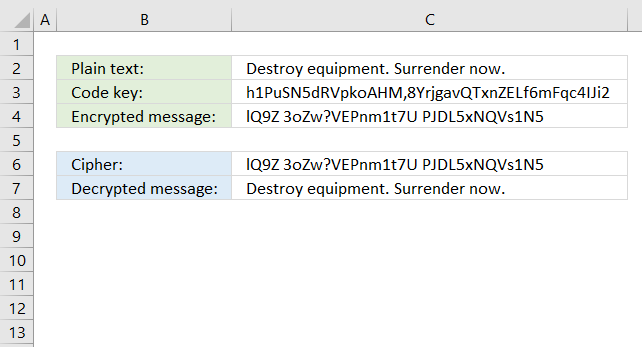The One-Time Pad cipher works just like the Vigenere cipher, however, the key must be random and not pseudo-random. It is not possible to crack a One-Time Pad cipher in theory, as long as the keys are only used once hence the name One-Time.

Formula in cell C4:

=TEXTJOIN(, TRUE, MID(I2, MOD(FIND(MID(C2, SEQUENCE(LEN(C2)), 1), I2)-1+FIND(MID(C3, MOD(SEQUENCE(LEN(C2))-1, LEN(C3))+1, 1), I2), LEN(I2))+1, 1))

Formula in cell C7:

=TEXTJOIN(,TRUE,MID(I2,MOD(FIND(MID(C6,SEQUENCE(LEN(C6)),1),I2)-1-FIND(MID(C3,MOD(SEQUENCE(LEN(C6))-1,LEN(C3))+1,1),I2),LEN(I2))+1,1))

## 18. Autokey cipher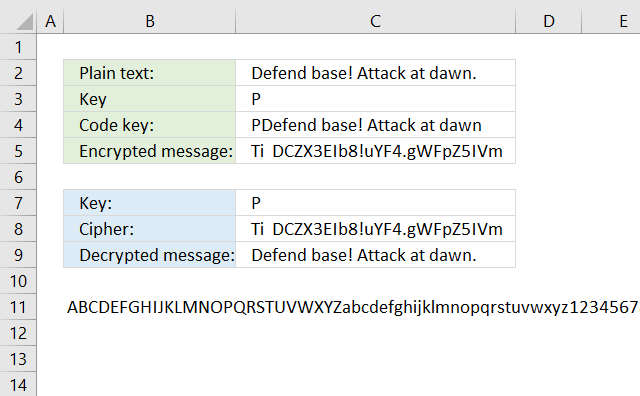The Autokey cipher is a Vigenere cipher, however, it uses the plaintext message concatenated with the real key. I have not yet figured out how to build a formula that can decrypt an Autokey cipher.

The LAMBDA function seems interesting, you can create a recursive function which seems handy in this case. It is still only available for Office Insiders.

Formula in cell C4:

=LEFT(C3&C2, LEN(C2))

Formula in cell C4:

=TEXTJOIN(, TRUE, MID(B11, MOD(FIND(MID(C2, SEQUENCE(LEN(C2)), 1), B11)-1+FIND(MID(C4, MOD(SEQUENCE(LEN(C2))-1, LEN(C4))+1, 1), B11), LEN(B11))+1, 1))

## 19. Homophonic substitution cipher (1)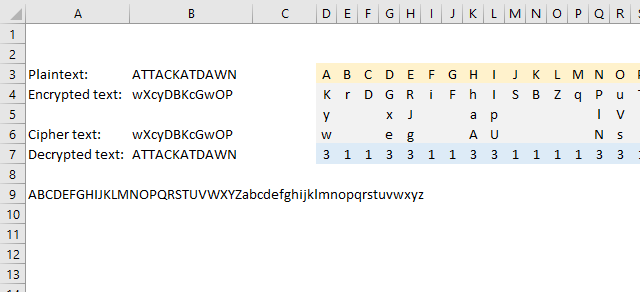The homophonic substitution cipher maps each character to a different character just like the simple substitution cipher, however, some characters are mapped to multiple characters which makes the cipher harder to break using frequency analysis.

I am using the following table to map characters, the formula picks a character randomly if more than one is entered.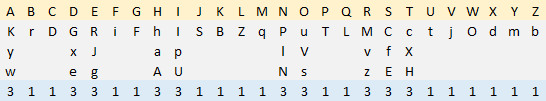Formula in cell B3:

=TEXTJOIN(, , INDEX(D4:AC6, RANDBETWEEN(1, INDEX(D7:AC7, MATCH(MID(B3, SEQUENCE(LEN(B3)), 1), D3:AC3, 0))), MATCH(MID(B3, SEQUENCE(LEN(B3)), 1), D3:AC3, 0)))

Formula in cell B7:

=TEXTJOIN(, , INDEX(D3:AC3, MOD(FIND(MID(B6, SEQUENCE(LEN(B6)), 1), TEXTJOIN(, FALSE, D4:AC6))-1, 26)+1))

## 20. Homophonic substitution cipher (2)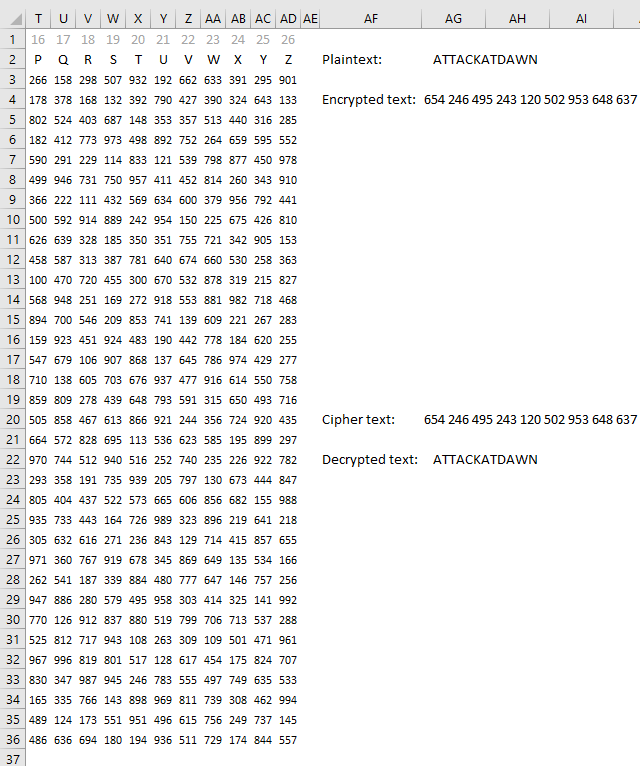This example is also a homophonic substitution cipher, however, a much larger table is used. It contains three unique digits per cell.

Formula in cell B3:

=TEXTJOIN(" ", FALSE, INDEX(E3#, RANDARRAY(LEN(AG2), , 1, 34, TRUE), MATCH(MID(AG2, SEQUENCE(LEN(AG2)), 1), E2#, 0)))

Formula in cell B7:

=TEXTJOIN(, TRUE, MID(C1, MOD(SEARCH(MID(AG20, SEQUENCE(LEN(SUBSTITUTE(AG20, " ", ""))/3, , , 4), 3)*1, TEXTJOIN(" ", TRUE, E3#))-1, 26*4)/4+1, 1))

## 21. XOR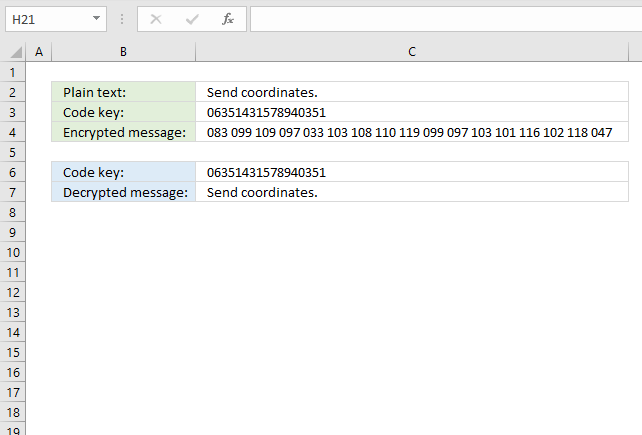The formula in cell C3 converts each character to ASCII code and then performs bitwise XOR between the code key and the ASCII code.

Formula in cell C3:

=TEXTJOIN(" ", FALSE, TEXT(BITXOR(CODE(MID(C2, SEQUENCE(LEN(C2)), 1)), MID(C3, SEQUENCE(LEN(C3)), 1)), "000"))

Formula in cell C7:

=TEXTJOIN(, TRUE, CHAR(BITXOR(MID(C4, SEQUENCE((LEN(C4)+1)/4, , , 4), 3)*1, MID(C6, SEQUENCE(LEN(C6)), 1))))

## 22. Four-square cipher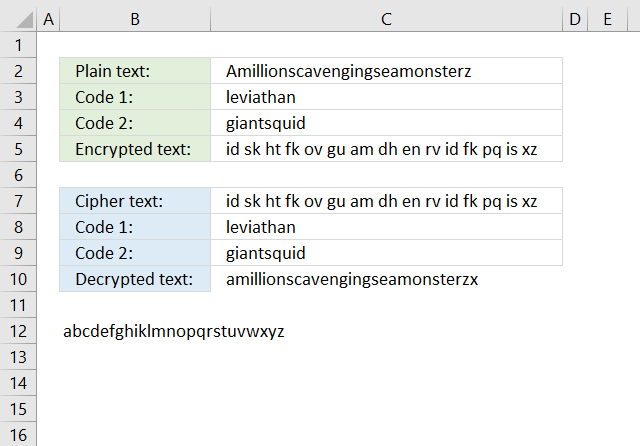The four-square cipher is a classic cipher from the 19 century that uses four grids to encrypt and decrypt messages. Two different code keys are used to change two of the grids.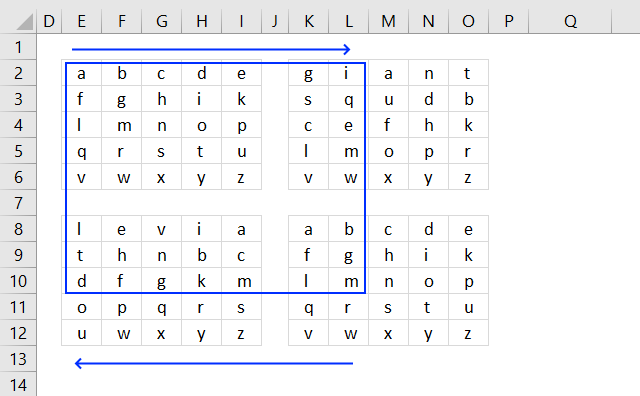The two first characters in the plain text are "a" and "m", find character "a" in the upper left grid, and find the character "m" in the lower right grid. They form a rectangle across all four grids, the two other characters in the opposite corners of the rectangle are the encrypted characters.

Note that the first code key is in the upper-right grid and the second code key is in the lower-left grid. The formulas below calculate the corresponding characters based on the plain text entered in cell C2.

Formula in cell C5:

=TEXTJOIN(" ", TRUE, INDEX(MID(TEXTJOIN(, FALSE, UNIQUE(MID(C4&B12, SEQUENCE(LEN(C4&B12)), 1))), SEQUENCE(5, 5), 1), ROUNDUP(SEARCH(MID(C2, SEQUENCE(ROUNDUP((LEN(C2))/2, 0), , , 2), 1), B12)/5, 0), MOD(SEARCH(MID(C2&"x", SEQUENCE(ROUNDUP((LEN(C2))/2, 0), , 2, 2), 1), B12)-1, 5)+1)&INDEX(MID(TEXTJOIN(, FALSE, UNIQUE(MID(C3&B12, SEQUENCE(LEN(C3&B12)), 1))), SEQUENCE(5, 5), 1), ROUNDUP(SEARCH(MID(C2&"x", SEQUENCE(ROUNDUP((LEN(C2))/2, 0), , 2, 2), 1), B12)/5, 0), MOD(SEARCH(MID(C2, SEQUENCE(ROUNDUP((LEN(C2))/2, 0), , , 2), 1), B12)-1, 5)+1))

Formula in cell C10:

=TEXTJOIN(, TRUE, INDEX(MID(B12, SEQUENCE(5, 5), 1), ROUNDUP(SEARCH(MID(SUBSTITUTE(C7, " ", ""), SEQUENCE(ROUNDUP((LEN(SUBSTITUTE(C7, " ", "")))/2, 0), , , 2), 1), TEXTJOIN(, TRUE, UNIQUE(MID(C9&B12, SEQUENCE(LEN(C9&B12)), 1))))/5, 0), MOD(SEARCH(MID(SUBSTITUTE(C7, " ", ""), SEQUENCE(ROUNDUP((LEN(SUBSTITUTE(C7, " ", "")))/2, 0), , 2, 2), 1), TEXTJOIN(, TRUE, UNIQUE(MID(C8&B12, SEQUENCE(LEN(C8&B12)), 1))))-1, 5)+1)&INDEX(MID(B12, SEQUENCE(5, 5), 1), ROUNDUP(SEARCH(MID(SUBSTITUTE(C7, " ", ""), SEQUENCE(ROUNDUP((LEN(SUBSTITUTE(C7, " ", "")))/2, 0), , 2, 2), 1), TEXTJOIN(, TRUE, UNIQUE(MID(C8&B12, SEQUENCE(LEN(C8&B12)), 1))))/5, 0), MOD(SEARCH(MID(SUBSTITUTE(C7, " ", ""), SEQUENCE(ROUNDUP((LEN(SUBSTITUTE(C7, " ", "")))/2, 0), , , 2), 1), TEXTJOIN(, TRUE, UNIQUE(MID(C9&B12, SEQUENCE(LEN(C9&B12)), 1))))-1, 5)+1))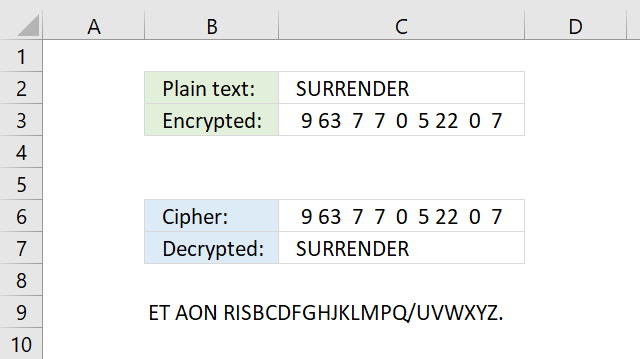The Straddling checkerboard converts letters to digits based on the table shown in the image below.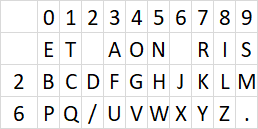The table contains numbers distributed above and to the left of a secret alphabet. For example, character "s" is in column 9 and the first row, the first row has no name (blank). The encrypted number for character "s" is 9.

Character "u" is in column 3 and row 6 the encrypted numbers for character "u" is therefore 63.

The straddling checkerboard is broken with frequency analysis.

Formula in cell C5:

=TEXTJOIN(" ", FALSE, INDEX(E3:E5, ROUNDUP(SEARCH(MID(C2, SEQUENCE(LEN(C2)), 1), B9)/10, 0))&INDEX(F2:O2, MOD(SEARCH(MID(C2, SEQUENCE(LEN(C2)), 1), B9)-1, 10)+1))

Formula in cell C10:

=TEXTJOIN(, TRUE, INDEX(F3:O5, MATCH(LEFT(MID(B11, SEQUENCE(ROUNDUP(LEN(B11)/3, 0), , , 3), 3), 1), E3:E5, 0), MATCH(MID(MID(B11, SEQUENCE(ROUNDUP(LEN(B11)/3, 0), , , 3), 3), 2, 1), F2:O2, 0)))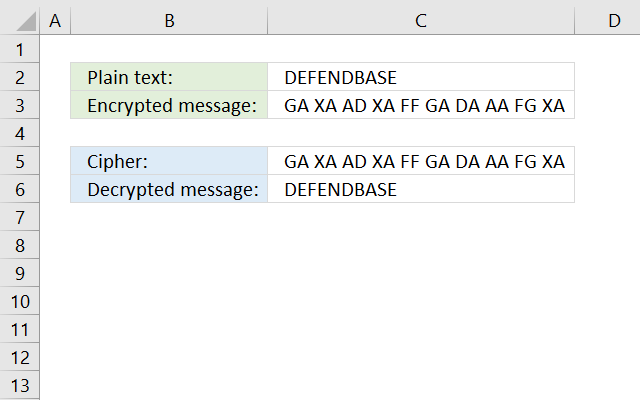The ADFGVX cipher converts each character to two different characters based on a grid, see image below.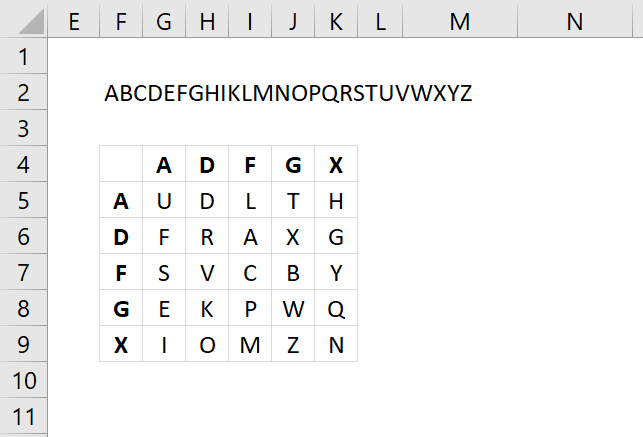A secret alphabet is used, see image above. The formulas below use the text string in cell F2, change this text string to create your own secret alphabet. Note that character "J" is not in the grid, if you have one in your plain text replace that character with I.

Formula in cell C3:

=TEXTJOIN(" ", TRUE, INDEX({"A", "D", "F", "G", "X"}, (MOD(SEARCH(MID(C2, SEQUENCE(LEN(C2)), 1), F2)-1, 5)+1))&INDEX({"A";"D";"F";"G";"X"}, (ROUNDUP(SEARCH(MID(C2, SEQUENCE(LEN(C2)), 1), F2)/5, 0))))

Replace array below {"A", "B", "C", "D", "E";"F", "G", "H", "IJ", "K";"L", "M", "N", "O", "P";"Q", "R", "S", "T", "U";"V", "W", "X", "Y", "Z"} with your secret alphabet. The order is important to successfully encrypt and decrypt messages.

Formula in cell C10:

=TEXTJOIN(, TRUE, INDEX({"A", "B", "C", "D", "E";"F", "G", "H", "IJ", "K";"L", "M", "N", "O", "P";"Q", "R", "S", "T", "U";"V", "W", "X", "Y", "Z"}, MATCH(MID(C5, SEQUENCE((LEN(C5)+1)/3, , , 3)+1, 1), {"A", "D", "F", "G", "X"}, 0), MATCH(MID(C5, SEQUENCE((LEN(C5)+1)/3, , , 3), 1), {"A", "D", "F", "G", "X"}, 0)))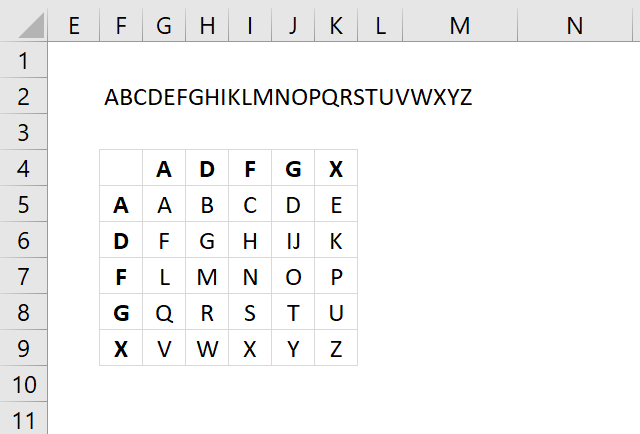## 25. Polybius square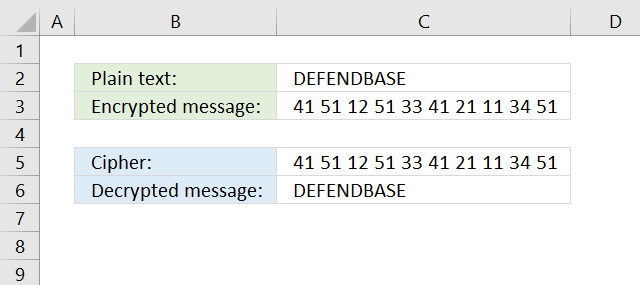The Polybius square encrypts a message by placing each character in a grid. The encrypted text is the column and row number of each character concatenated to a long string.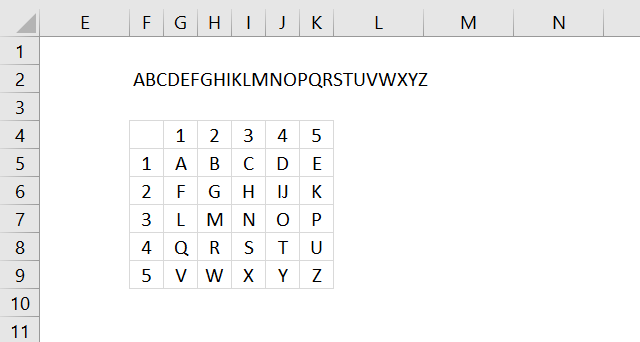Formula in cell C3:

=TEXTJOIN(" ", TRUE, (MOD(SEARCH(MID(C2, SEQUENCE(LEN(C2)), 1), F2)-1, 5)+1)&(ROUNDUP(SEARCH(MID(C2, SEQUENCE(LEN(C2)), 1), F2)/5, 0)))

Formula in cell C10:

=TEXTJOIN(, TRUE, MID(F2, MID(C5, SEQUENCE((LEN(C5)+1)/3, , , 3), 1)+(MID(C5, SEQUENCE((LEN(C5)+1)/3, , , 3)+1, 1)-1)*5, 1))

## 26. Nihilist cipher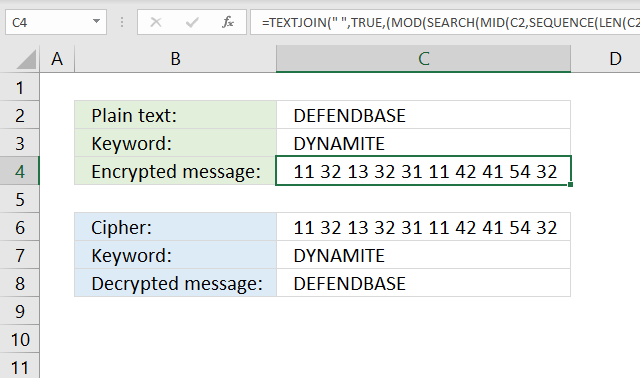Formula in cell C3:

=TEXTJOIN(" ", TRUE, (MOD(SEARCH(MID(C2, SEQUENCE(LEN(C2)), 1), F3)-1, 5)+1)&(ROUNDUP(SEARCH(MID(C2, SEQUENCE(LEN(C2)), 1), F3)/5, 0)))

Formula in cell C10:

=TEXTJOIN(, TRUE, MID(F3, MID(C6, SEQUENCE((LEN(C6)+1)/3, , , 3), 1)+(MID(C6, SEQUENCE((LEN(C6)+1)/3, , , 3)+1, 1)-1)*5, 1))

## 27. Bifid cipher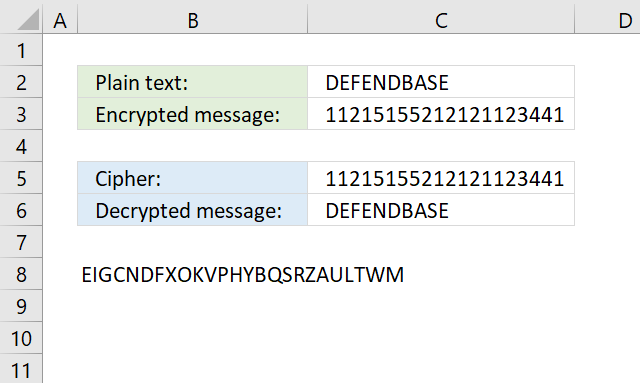Formula in cell C3:

=TEXTJOIN(, TRUE, (MOD(SEARCH(MID(C2, SEQUENCE(LEN(C2)), 1), B8)-1, 5)+1))&TEXTJOIN(, TRUE, (ROUNDUP(SEARCH(MID(C2, SEQUENCE(LEN(C2)), 1), B8)/5, 0)))

Formula in cell C6:

=TEXTJOIN(, TRUE, INDEX(MID(B8, SEQUENCE(5, 5), 1), MID(RIGHT(C5, LEN(C5)/2), SEQUENCE(LEN(C5)/2), 1), MID(LEFT(C5, LEN(C5)/2), SEQUENCE(LEN(C5)/2), 1)))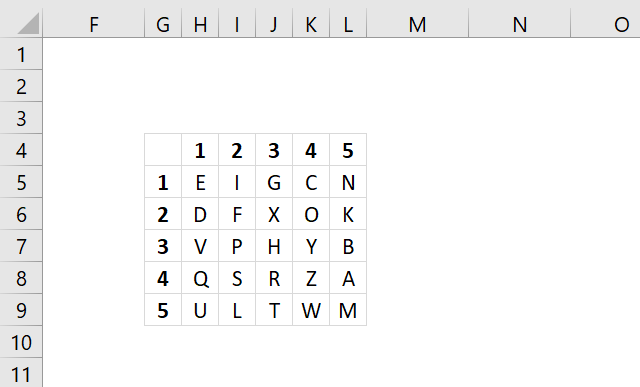## 29. Frequency analysis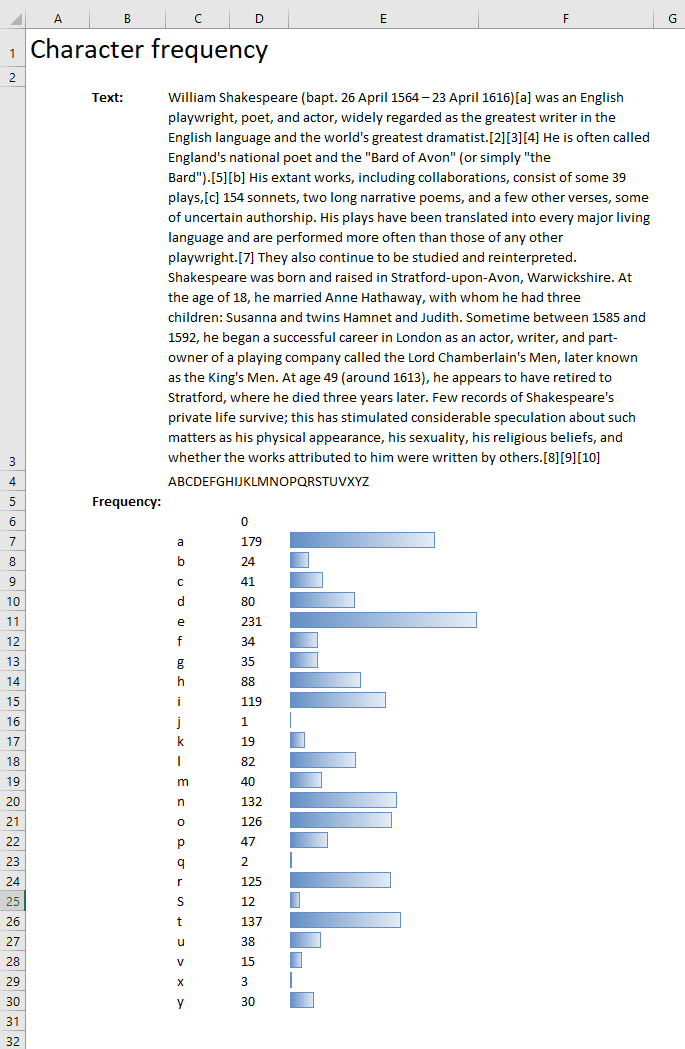Formula in cell C6:

=SORT(UNIQUE(IF(ISNUMBER(SEARCH(MID(C3, SEQUENCE(LEN(C3)), 1), C4)), MID(C3, SEQUENCE(LEN(C3)), 1), "")))

Formula in cell D6:

=LEN(\$C\$3)-LEN(SUBSTITUTE(\$C\$3, C6#, ""))